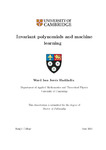## Invariant polynomials and machine learning

##### Repository DOIThesis
##### Abstract

In this thesis, we demonstrate the benefit of incorporating our knowledge of the symmetries of certain systems into the machine learning methods used to model them. By borrowing the necessary tools from commutative algebra and invariant theory, we construct systematic methods to obtain sets of invariant variables that describe two such systems: particle physics and physical chemistry. In both cases, our systems are described by a collection of vectors. In the former, these vectors represent the particle momenta in collision events where our system is Lorentz- and permutation-invariant. In the latter, the vectors represent the positions of atoms in molecules (or lattices) where our systems are Euclidean- and permutation-invariant.

We start by focusing on the algebras of invariant polynomials in these vectors and design systematic methods to obtain sets of generating variables. To do so, we build on two theorems of Weyl which tell us that the algebra of orthogonal group-invariant polynomials in $n$ $d$-dimensional vectors is generated by the dot products and that the redundancies which arise when $n>d$ are generated by the $\left(d+1\right)$-minors of the $n×n$ matrix of dot products. We prove the equivalent theorems for the algebra of polynomials invariant under the Euclidean group which provide us with a similar set of variables for describing molecular properties. We also extend these results to include the action of an arbitrary permutation group $P\subset Sn$ on the vectors. Doing so furnishes us with sets of variables for describing molecules with identical atoms or scattering processes involving identical particles, such as $pp\to jjj$, for which we provide an explicit minimal set of Lorentz- (and parity-) and permutation-invariant generators. Additionally, we use the Cohen-Macaulay structure of the Lorentz-invariant algebra to provide a more direct characterisation in terms of a Hironaka decomposition. Although such a characterisation would na"ively not be expected for the Euclidean-invariant algebra (since the Euclidean group is not linearly reductive), we show that it is in fact also Cohen-Macaulay by establishing that it is isomorphic to another Cohen-Macaulay algebra, namely the Lorentz-invariant algebra in one vector fewer. Among the benefits of Hironaka decompositions is that they can be generalized straightforwardly to when parity is not a symmetry and to cases where a permutation group acts on the particles. In the first non-trivial case, $n=d+1$, we give a homogeneous system of parameters that is valid for the action of an arbitrary permutation symmetry and make a conjecture for the full Hironaka decomposition in the case without permutation symmetry.

Armed with the knowledge of characterising invariant algebras, we then discuss why they are indeed sufficient for our purposes. More precisely, we discuss and prove some approximation theorems which allow us to use the generators of invariant algebras to approximate continuous invariant functions in machine learning algorithms in general and in neural networks specifically. Finally, we implement these variables in neural networks applied to regression tasks to test the efficiency improvements. We perform our testing for a variety of hyperparameter choices and find an overall reduction of the loss on training data and a significant reduction of the loss on validation data. For a different approach on quantifying the performance of these neural networks, we treat the problem from a Bayesian inference perspective and employ nested sampling techniques to perform model comparison. Beyond a certain network size, we find that networks utilising Hironaka decompositions perform the best.

2021-06-04